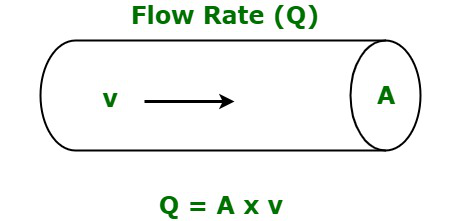# Flow Rate Formula

• Last Updated : 21 Jun, 2022

Flow rate is the measurement of how much liquid travels in a given length of time, as the name implies. Its current is also affected by the pipe’s diameter. The flow rate of a fluid is its ability to flow from one point to another.

### What is Flow Rate?

The flow rate of a liquid is defined as the measure of the volume of liquid that moves in a certain amount of time. It is the amount of fluid that moves through a given area in a given amount of time. It can be interpreted as the velocity of the liquid for a cross-sectional area of the pipe.It varies directly with the area of the pipe and the velocity with which the liquid flows in a pipe.

• It is denoted by the symbol Q.
• Its standard unit of measurement is cubic meters per second (m3/s) and the dimensional formula is given by [M0L3T-1].

### Flow Rate Formula

The formula for flaw rate is given as,

Q = Av

where,

• Q is the flow rate,
• A is area of cross section of pipe,
• v is the velocity of the fluid.

The Flow rate Q can also be defined to be the volume of fluid passing by some position through an area during a period of time.

Q = V / t

where,

• V is the volume of fluid and
• t is the period of time taken.

### Sample Problems

Problem 1: Calculate the flow rate of liquid moving in a pipe of cross-section 20 m2 at a velocity of 10 m/s.

Solution:

We have,

A = 20

v = 10

Using the formula we have,

Q = Av

= 20 (10)

= 200 m3/s

Problem 2: Calculate the flow rate of liquid moving in a pipe of cross-section 15 m2 at a velocity of 12 m/s.

Solution:

We have,

A = 15

v = 12

Using the formula we have,

Q = Av

= 15 (12)

= 180 m3/s

Problem 3: Calculate the flow rate of a liquid moving in a pipe of radius 2 m at a velocity of 10 m/s.

Solution:

We have,

r = 2

v = 10

Calculate the cross section area.

A = πr2

= 3.14 (2) (2)

= 12.56 m2

Using the formula we have,

Q = Av

= 12.56 (10)

= 125.6 m3/s

Problem 4: Calculate the velocity of the liquid if its flow rate is 100 m3/s in a pipe of cross-section 50 m2.

Solution:

We have,

Q = 100

A = 50

Using the formula we have,

Q = Av

or

v = Q/A

= 100/50

= 2 m/s

Problem 5: Calculate the velocity of the liquid if its flow rate is 144 m3/s in a pipe of cross-section 36 m2.

Solution:

We have,

Q = 144

A = 36

Using the formula we have,

Q = Av

or

v = Q/A

= 144/36

= 4 m/s

Problem 6: Calculate the area of the cross-section if its flow rate is 90 m3/s moving at a velocity of 15 m/s.

Solution:

We have,

Q = 90

v = 15

Using the formula we have,

Q = Av

or A = Q/v

= 90/15

= 6 m2

Problem 7: Calculate the area of the cross-section if its flow rate is 120 m3/s moving at a velocity of 45 m/s.

Solution:

We have,

Q = 120

v = 45

Using the formula we have,

Q = Av

or

A = Q/v

= 120/45

= 2.66 m2

My Personal Notes arrow_drop_up